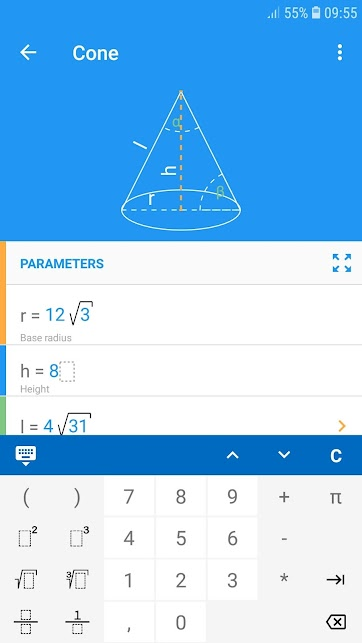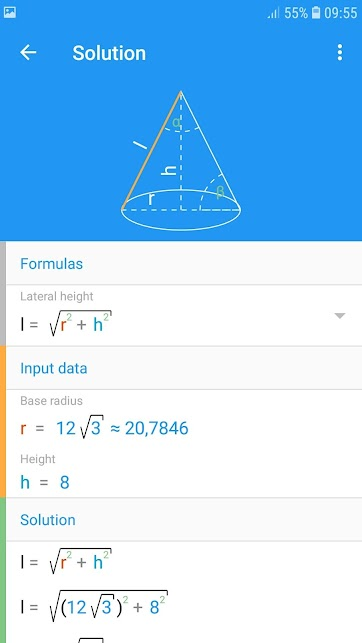# Math Studio [Paid] APK v2.32 build 95

#### Blackvol

##### A simple man
Staff member
Blackvol submitted a new resource:

Math Studio [Paid] APK - All in one. Step by step solution.All in one. Step by step solution.
This app includes a lot of mathematics topics such as geometry, analytic geometry, equations and inequalities, quadratic function, linear function, linear system, circle equation, math sequences...

•Kessler

#### Blackvol

##### A simple man
Staff member
Blackvol updated Math Studio [Paid] APK with a new update entry:

Math Studio APK

All in one. Step by step solution.
This app includes a lot of mathematics topics such as geometry, analytic geometry, equations and inequalities, quadratic function, linear function, linear system, circle equation, math sequences, algebra, vectors.
It also contains units calculator.

GEOMETRY
- Triangles: equilateral triangle, right triangle, isosceles triangle, 30-60-90
- Quadrilaterals: square, rectangle, rhombus, parallelogram, trapezoid, right trapezoid, isosceles trapezoid, kite
-...

Read the rest of this update entry...

•Kessler

### Members online

No members online now.